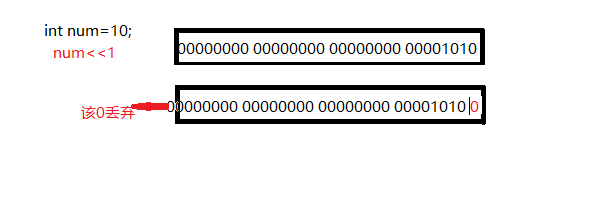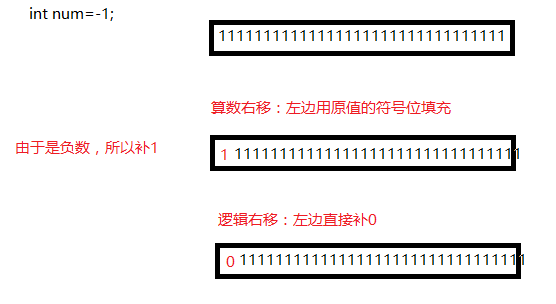# c语言：操作符小结

2017-11-15 09:53 89 查看

1，算术操作符

+ - * / %

1. 除了%操作符之外，其他的几个操作符可以作用于整数和浮点数。

2. 对于/操作符如果两个操作数都为整数，执行整数除法。而只要有浮点数执行的就是浮点数除法。

3. %操作符的两个操作数必须为整数。返回的是整除之后的余数。

2，移位操作符

<< 左移操作符 >> 右移操作符

1. 逻辑移位 左边用0填充，右边丢弃

2. 算术移位 左边用原该值的符号位填充，右边丢弃3，位操作符

& //按位与

| //按位或

^ //按位异或

4，赋值操作符

```int weight = 120;//体重
weight = 89;//不满意就赋值
double salary = 10000.0;
salary = 20000.0;//使用赋值操作符赋值。```

```int a = 10;
int x = 0;
int y = 20;
a = x = y+1;//连续赋值  ，但不符合我们的编码规范
//换一种写法
x = y+1;
a = x; //这样的写法更符合我们的编码规范并且易于观察的调试```

5，复合赋值符

+=

-=

%=

&=

|=

^=

```int x = 10;
x = x+10;
x+=10;//复合赋值```

6，单目操作符

! 逻辑反操作

- 负值

+ 正值

& 取地址

sizeof 操作数的类型长度（以字节为单位）

~ 对一个数的二进制按位取反

– 前置、后置–

++ 前置、后置++

* 间接访问操作符(解引用操作符) 只能对指针解引用

(类型) 强制类型转换

```int a;
//sizeof（数组名），数组名表示整个数组，表达式求的是整个数组的大小，单位是字节
//&a---数组名表示整个数组，表示求的是整个数组的地址
//初次之外，所有的数组名出现都表示首元素的地址。```

7,关系操作符

’ > >=

< <= ‘//此处操作符无引号，只是为了方便说明

!= 用于测试“不相等”

== 用于测试“相等”

8,逻辑操作符

&& 逻辑与

|| 逻辑或

1&2—–>0

1&&2—->1

1|2—–>3

1||2—->1

9,条件操作符

exp1 ? exp2 : exp3

```int a,b,c;
a=b>c?b:c//将b和c中较大者赋给a```

10,逗号表达式

exp1, exp2, exp3, …expN

```int a = 1;
int b = 2;
int c = (a>b, a=b+10, a, b=a+1);//c=14```

11,下标引用、函数调用和结构成员

1. [ ] 操作数：一个数组名 + 一个索引值

```int arr;//创建数组
arr = 10;//实用下标引用操作符。
[ ]的两个操作数是arr和9。```

2 () 函数调用操作符 接受一个或者多个操作数：第一个操作数是函数名，剩余的操作数就是传递给函数 的参数。

```void test1()
{
printf("hehe\n");
}
void test2(const char *str)
{
printf("%s\n", str);
}
int main()
{
test1();            //实用（）作为函数调用操作符。
test2("welcome");//实用（）作为函数调用操作符。
return 0;
}```

3 访问一个结构的成员 . 结构体.成员名 -> 结构体指针->成员名

```#include <stdio.h>
struct Stu
{
char name;
int age;
char sex;
double score;
}；
void set_age1(struct Stu stu)
{
stu.age = 18;
}
void set_age2(struct Stu* pStu)
{
pStu->age = 18;//结构成员访问
}
int main()
{
struct Stu stu;
struct Stu* pStu = &stu;//结构成员访问
stu.age = 20;//结构成员访问
set_age1(stu);
pStu->age = 20;//结构成员访问
set_age2(pStu);
return 0;
}```